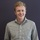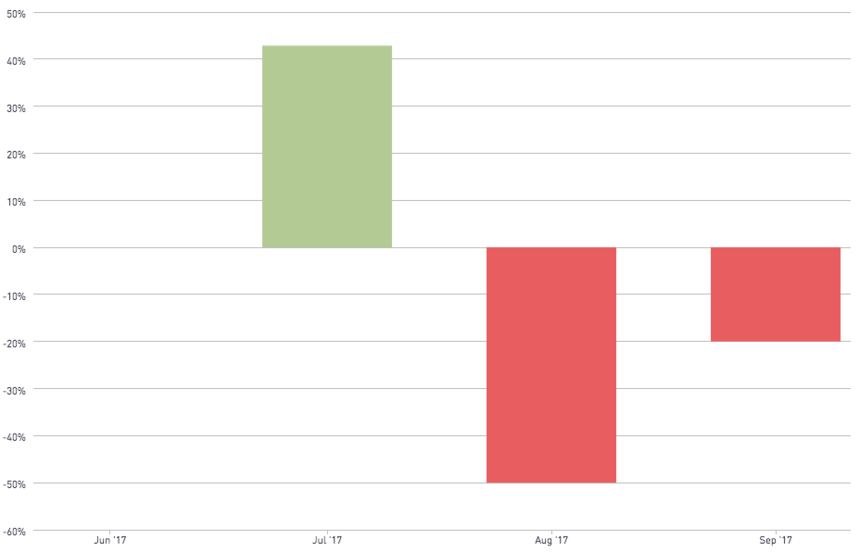# Chart Type - Period over Period Change

An important analytical technique is to look at the rate of change over time. An easy graph to investigate this is a bar chart with the period over period change in percent or value. In this snippet, you can calculate the change for various time periods leveraging the built-in aggregation functions. If the user wants to use the aggregation filter, they can pass “aggregation” in the aggregation parameter. The “direction” field is for series to allow red/green or other types of color schemes to quickly indicate positives and negatives.Base: Bar Chart

• With period_over_period_direction as series
• ‘positive’ color = #b5ca92
• ‘negative’ color = #df5c5c

Name: period_over_period_change(table,field,date)

```select
date
, [field]
, case
when lag([field]) over(order by date) is null
then null
else 1.0 *[field] / lag([field]) over(order by date) - 1
end as period_over_period_change_perc
, case
when lag([field]) over(order by date) is null
then null
else [field] - lag([field]) over(order by date) - 1
end as period_over_period_change_value
, case
when lag([field]) over(order by date) is null
then null
else (case when lag([field]) over(order by date) > [field] then 'negative' else 'positive' end)
end as period_over_period_change_direction
from
(
select
[field]
, [date] as date
from
[table]
)```